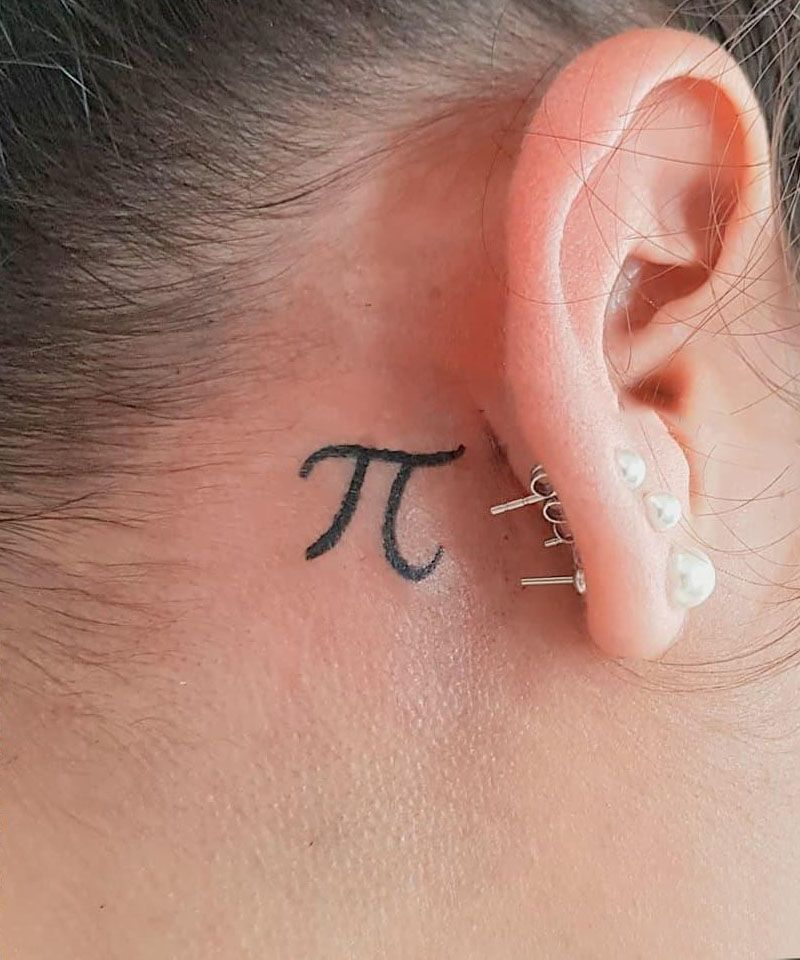# 30 Unique PI Tattoos You Can Copy

The most common figure in the world may be round, such as falling raindrops, the cross-section of a tree trunk, the flower center of a flower, the sun and moon overhead, and various stars and planets observed under various high-end astronomical telescopes.

The PI we know today is usually expressed by the Greek letter π. It is the ratio of the circumference of a circle to its diameter, and also the ratio of its area to the square of its radius. It can be used to calculate the circumference, area, volume of a sphere and so on.

Mathematicians believe that Pi is actually an irrational number, in other words, an infinite non cyclic decimal. It has no numerical law and will be arranged in a relatively disorderly way all the time.Source: @__.space.babe.art.__